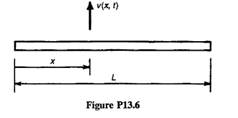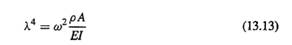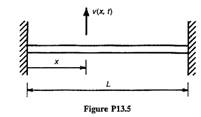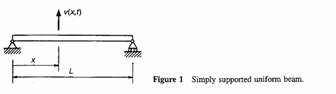### Create an Account

Home / Questions / Flight vehicles such as airplanes and rockets are unconstrained and therefore they can und...

# Flight vehicles such as airplanes and rockets are unconstrained and therefore they can undergo rigid body motion The free-free uniform beam gives some insight into the behavior

Flight vehicles such as airplanes and rockets are unconstrained, and therefore they can undergo rigid body motion. The free-free uniform beam gives some insight into the behavior of such free-free structures. Consider transverse vibration of the uniform beam (El = constant, pA = constant) shown in Fig. P13.6. (a) Show that the beam has two zero-frequency (i.e., rigid-body) modes, one mode in translation and one in rotation. (b) Determine the characteristic equation governing the nonzero frequencies. Your answer should contain the parameters λ and L. where λ is defined by Eq. 13.13. (Note that this is the same equation as obtained in Problem 13.5 for a uniform clamped-clamped beam.) (c) Determine an expression for the fundamental nonzero frequency of the beam (ω3 rad/s). (Note: For the rigid body modes, ω1, = ω2 = 0.) (d) Determine an expression for the fundamental flexible transverse-vibration mode shape, φ3 (x ), and use M a t l a b , or another computer program, to plot the fundamental flexible mode. Scale the mode so that its maximum value is 1.0.Problem 13.5

Consider transverse vibration of the uniform clamped-clamped beam shown in Fig. P13.5.&#39; (a) Determine the characteristic equation for transverse vibration. Your answer should contain the parameters λ and L, where λ is defined by Eq. 13.13. (b) Determine an expression for the fundamental transverse-vibration frequency of the beam (tus rad/s), (c) Determine an expression for the fundamental transverse-vibration mode shape, φ1(x) and use M a t l a b , or another computer program, to plot the fundamental mode. Scale the mode so that its maximum value is 1.0. (d) Using the results of Example 13.2, compare the fundamental frequency of the clamped-clamped beam with that of a simply supported beam. What is the effect of adding the slope constraints to the beam at x = 0 and x = L?Example 13.2

Determine the natural frequencies and natural modes of a uniform beam that is simply supported at both ends, as shown in Fig. 1.May 19 2020 View more View LessSubscribe To Get Solution